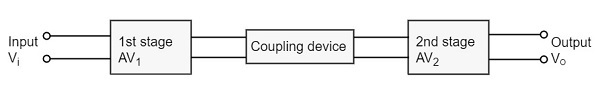# Multi-Stage Transistor Amplifier

Advertisements

In practical applications, the output of a single state amplifier is usually insufficient, though it is a voltage or power amplifier. Hence they are replaced by Multi-stage transistor amplifiers.

In Multi-stage amplifiers, the output of first stage is coupled to the input of next stage using a coupling device. These coupling devices can usually be a capacitor or a transformer. This process of joining two amplifier stages using a coupling device can be called as Cascading.

The following figure shows a two-stage amplifier connected in cascade.The overall gain is the product of voltage gain of individual stages.

$$A_V = A_{V1} \times A_{V2} = \frac{V_2}{V_1} \times \frac{V_0}{V_2} = \frac{V_0}{V_1}$$

Where AV = Overall gain, AV1 = Voltage gain of 1st stage, and AV2 = Voltage gain of 2nd stage.

If there are n number of stages, the product of voltage gains of those n stages will be the overall gain of that multistage amplifier circuit.

### Purpose of coupling device

The basic purposes of a coupling device are

• To transfer the AC from the output of one stage to the input of next stage.

• To block the DC to pass from the output of one stage to the input of next stage, which means to isolate the DC conditions.

## Types of Coupling

Joining one amplifier stage with the other in cascade, using coupling devices form a Multi-stage amplifier circuit. There are four basic methods of coupling, using these coupling devices such as resistors, capacitors, transformers etc. Let us have an idea about them.

### Resistance-Capacitance Coupling

This is the mostly used method of coupling, formed using simple resistor-capacitor combination. The capacitor which allows AC and blocks DC is the main coupling element used here.

The coupling capacitor passes the AC from the output of one stage to the input of its next stage. While blocking the DC components from DC bias voltages to effect the next stage. Let us get into the details of this method of coupling in the coming chapters.

### Impedance Coupling

The coupling network that uses inductance and capacitance as coupling elements can be called as Impedance coupling network.

In this impedance coupling method, the impedance of coupling coil depends on its inductance and signal frequency which is jwL. This method is not so popular and is seldom employed.

### Transformer Coupling

The coupling method that uses a transformer as the coupling device can be called as Transformer coupling. There is no capacitor used in this method of coupling because the transformer itself conveys the AC component directly to the base of second stage.

The secondary winding of the transformer provides a base return path and hence there is no need of base resistance. This coupling is popular for its efficiency and its impedance matching and hence it is mostly used.

### Direct Coupling

If the previous amplifier stage is connected to the next amplifier stage directly, it is called as direct coupling. The individual amplifier stage bias conditions are so designed that the stages can be directly connected without DC isolation.

The direct coupling method is mostly used when the load is connected in series, with the output terminal of the active circuit element. For example, head-phones, loud speakers etc.

## Role of Capacitors in Amplifiers

Other than the coupling purpose, there are other purposes for which few capacitors are especially employed in amplifiers. To understand this, let us know about the role of capacitors in Amplifiers.

### The Input Capacitor Cin

The input capacitor Cin present at the initial stage of the amplifier, couples AC signal to the base of the transistor. This capacitor Cin if not present, the signal source will be in parallel to resistor R2 and the bias voltage of the transistor base will be changed.

Hence Cin allows, the AC signal from source to flow into input circuit, without affecting the bias conditions.

### The Emitter By-pass Capacitor Ce

The emitter by-pass capacitor Ce is connected in parallel to the emitter resistor. It offers a low reactance path to the amplified AC signal.

In the absence of this capacitor, the voltage developed across RE will feedback to the input side thereby reducing the output voltage. Thus in the presence of Ce the amplified AC will pass through this.

### Coupling Capacitor CC

The capacitor CC is the coupling capacitor that connects two stages and prevents DC interference between the stages and controls the operating point from shifting. This is also called as blocking capacitor because it does not allow the DC voltage to pass through it.

In the absence of this capacitor, RC will come in parallel with the resistance R1 of the biasing network of the next stage and thereby changing the biasing conditions of the next stage.

## Amplifier Consideration

For an amplifier circuit, the overall gain of the amplifier is an important consideration. To achieve maximum voltage gain, let us find the most suitable transistor configuration for cascading.

### CC Amplifier

• Its voltage gain is less than unity.
• It is not suitable for intermediate stages.

### CB Amplifier

• Its voltage gain is less than unity.
• Hence not suitable for cascading.

### CE Amplifier

• Its voltage gain is greater than unity.
• Voltage gain is further increased by cascading.

The characteristics of CE amplifier are such that, this configuration is very suitable for cascading in amplifier circuits. Hence most of the amplifier circuits use CE configuration.

In the subsequent chapters of this tutorial, we will explain the types of coupling amplifiers.

Advertisements